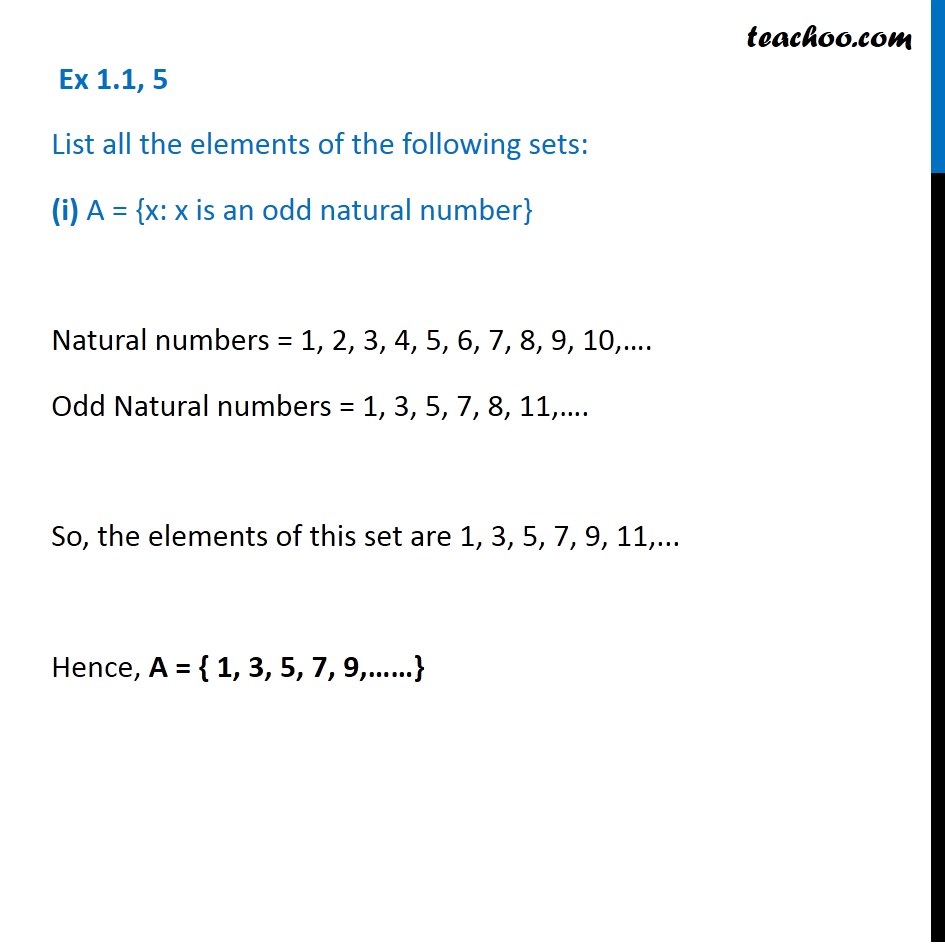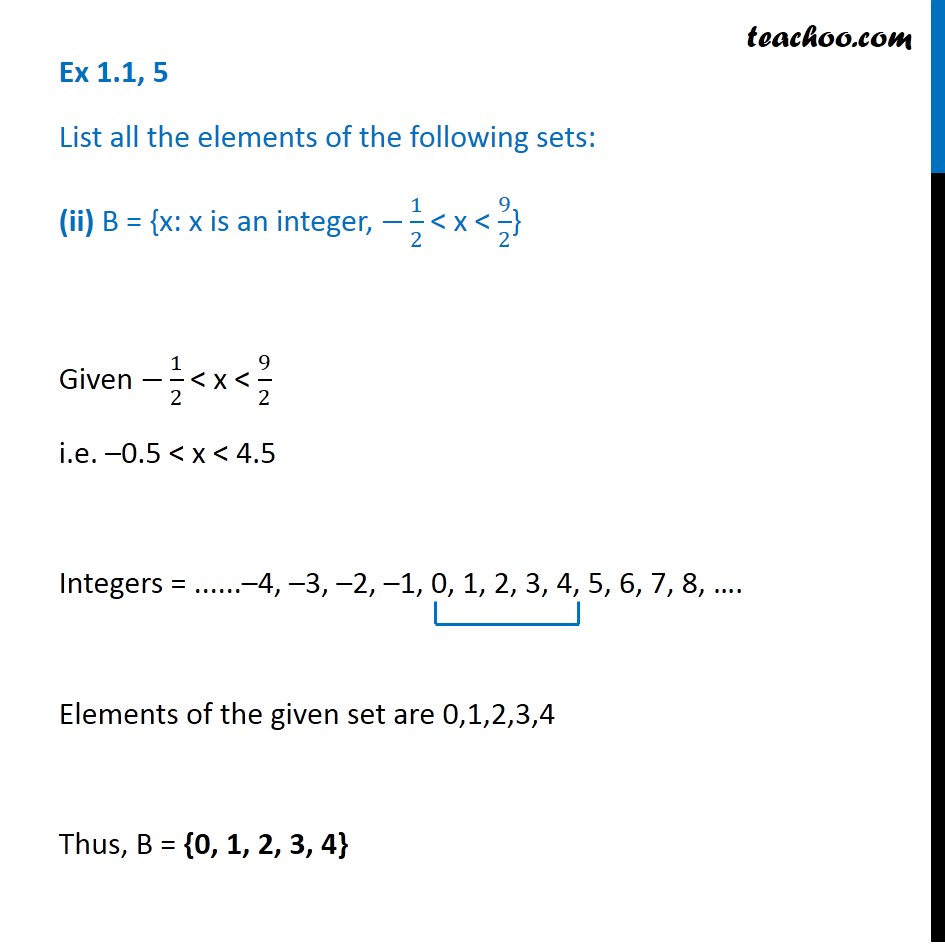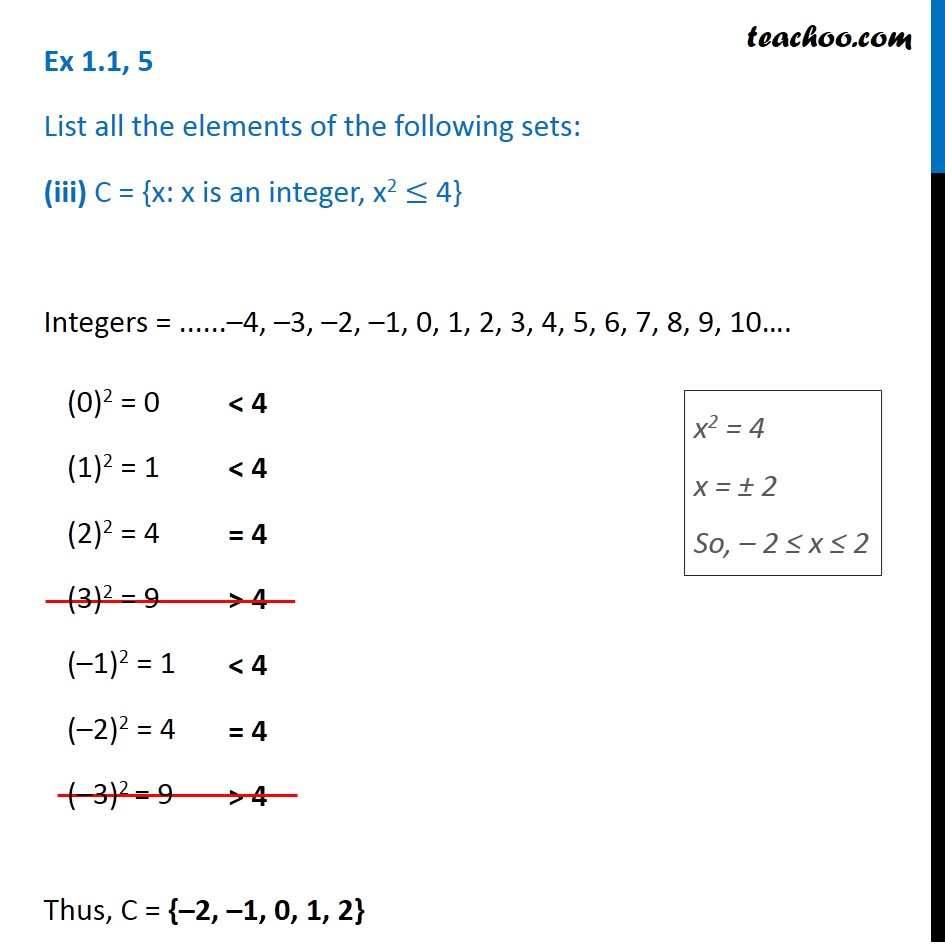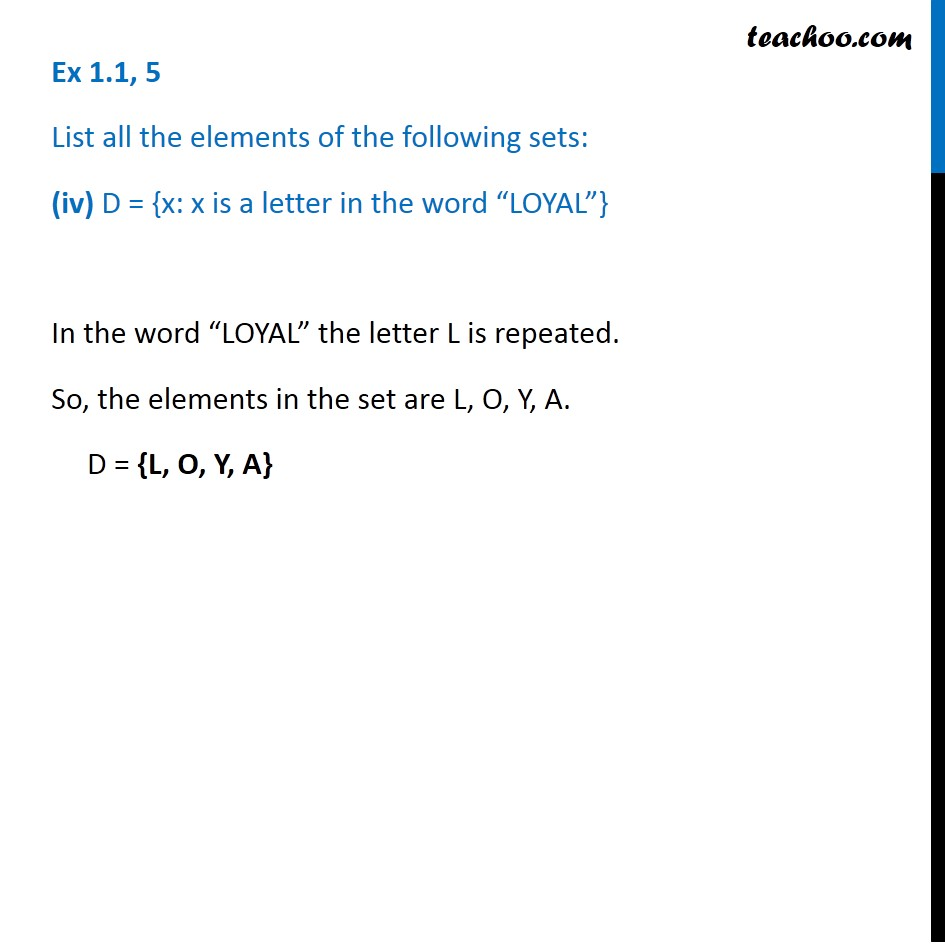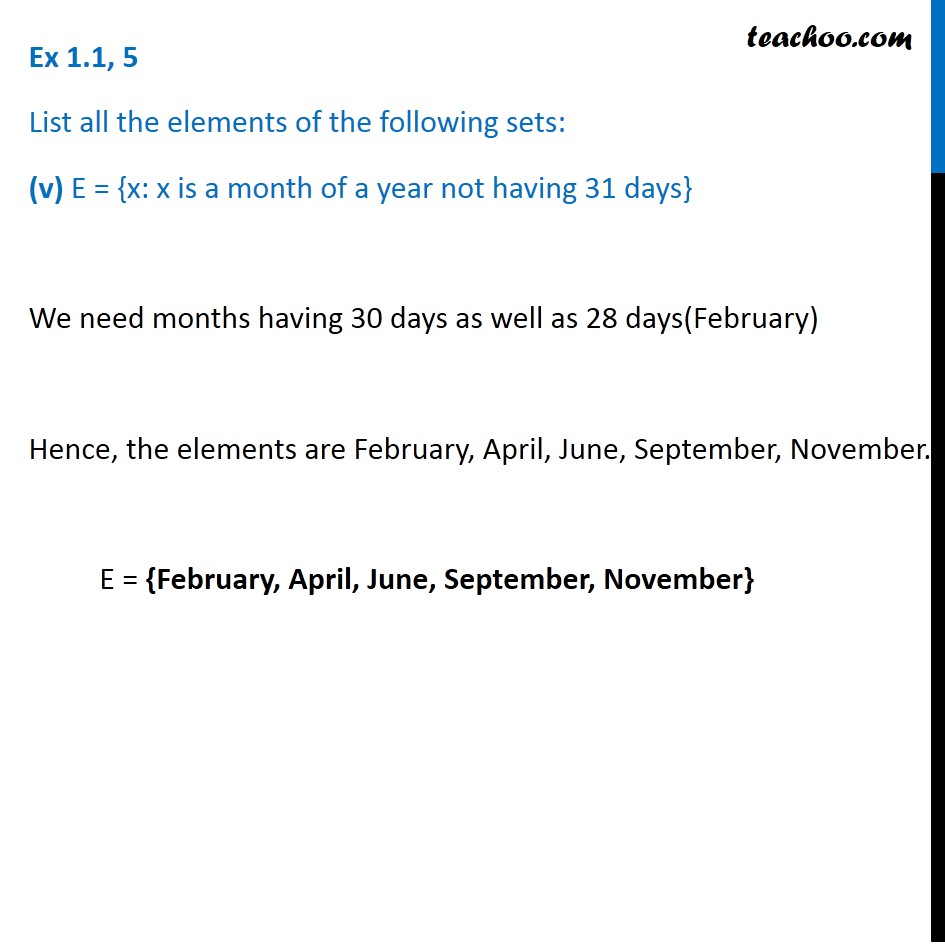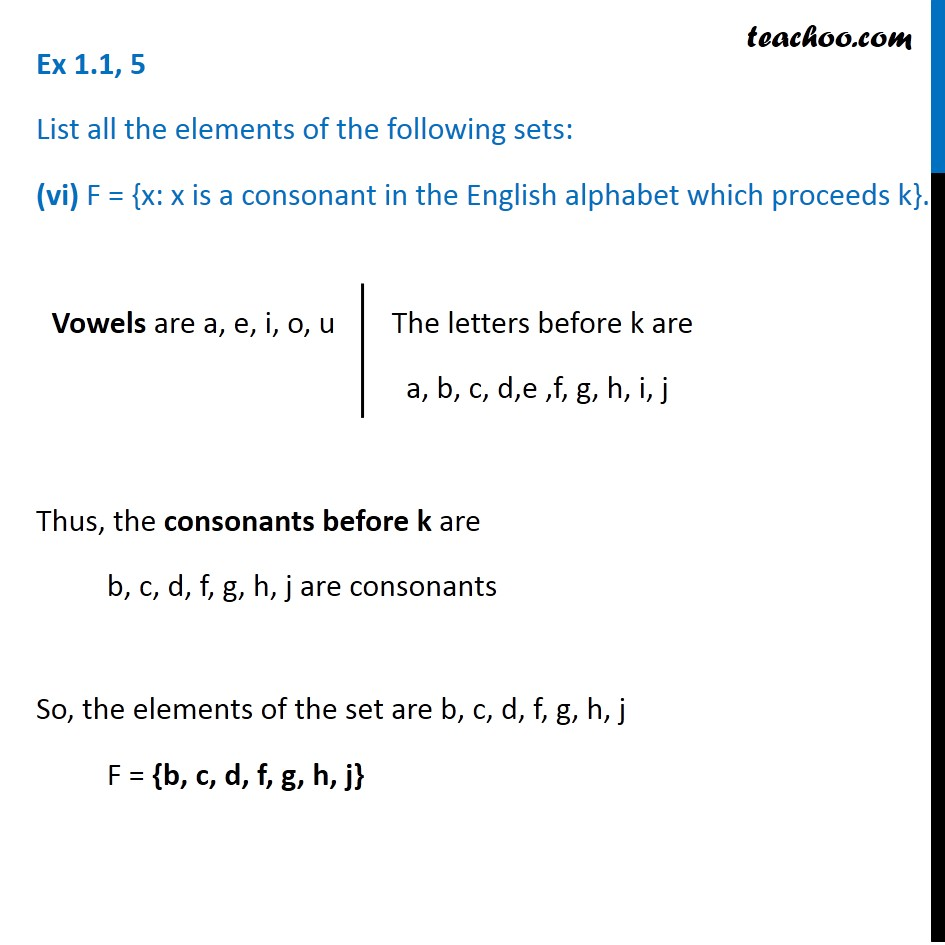1. Chapter 1 Class 11 Sets
2. Concept wise
3. Depicition of sets - Roster form

Transcript

Ex 1.1, 5 List all the elements of the following sets: (i) A = {x: x is an odd natural number} Natural numbers = 1, 2, 3, 4, 5, 6, 7, 8, 9, 10,…. Odd Natural numbers = 1, 3, 5, 7, 8, 11,…. So, the elements of this set are 1, 3, 5, 7, 9, 11,... Hence, A = { 1, 3, 5, 7, 9,……} Ex 1.1, 5 List all the elements of the following sets: (ii) B = {x: x is an integer, −1/2 < x < 9/2} Given −1/2 < x < 9/2 i.e. –0.5 < x < 4.5 Integers = ......–4, –3, –2, –1, 0, 1, 2, 3, 4, 5, 6, 7, 8, …. Elements of the given set are 0,1,2,3,4 Thus, B = {0, 1, 2, 3, 4} Ex 1.1, 5 List all the elements of the following sets: (iii) C = {x: x is an integer, x2 ≤ 4} Integers = ......–4, –3, –2, –1, 0, 1, 2, 3, 4, 5, 6, 7, 8, 9, 10…. Thus, C = {–2, –1, 0, 1, 2} (0)2 = 0 (1)2 = 1 (2)2 = 4 (3)2 = 9 (–1)2 = 1 (–2)2 = 4 (–3)2 = 9 x2 = 4 x = ± 2 So, – 2 ≤ x ≤ 2 Ex 1.1, 5 List all the elements of the following sets: (iv) D = {x: x is a letter in the word “LOYAL”} In the word “LOYAL” the letter L is repeated. So, the elements in the set are L, O, Y, A. D = {L, O, Y, A} Ex 1.1, 5 List all the elements of the following sets: (v) E = {x: x is a month of a year not having 31 days} We need months having 30 days as well as 28 days(February) Hence, the elements are February, April, June, September, November. E = {February, April, June, September, November} Ex 1.1, 5 List all the elements of the following sets: (vi) F = {x: x is a consonant in the English alphabet which proceeds k}. Thus, the consonants before k are b, c, d, f, g, h, j are consonants So, the elements of the set are b, c, d, f, g, h, j F = {b, c, d, f, g, h, j} Vowels are a, e, i, o, u The letters before k are a, b, c, d,e ,f, g, h, i, j

Depicition of sets - Roster form